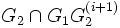# Normalizing group intersection problem

This article describes a group-finding problem of the following form: two groups are given (by means of their generating sets) and we need to find a generating set for their intersection

This article describes a problem in the setup where the group(s) involved is/are defined by means of an embedding in a suitable universe group (such as a linear or a permutation group) -- viz in terms of generators described as elements sitting inside this universe group

## Description

### Given data

Our universe is some group$U$ (such as a linear group or a permutation group) in which products and inverses can be readily computed.

Two groups$G_1$ and$G_2$ in$U$ are specified by means of their generating sets$A_1$ and$A_2$. We are also given that$G_1$ is a normalizing subgroup for$G_2$, viz every element of$G_1$ normalizes the subgroup$G_2$ of$U$.

### Goal

We are required to determine a generating set for$G_1$$G_2$.

## Relation with other problems

### Problems it reduces to

Actually, the solution that we describe for this problem works for the more general mutually permuting group intersection problem.

## Solution

### The underlying descending chain

We have the goal of computing$G_1 \cap G_2$. First, consider the descending chain of subgroups:$G_2 \geq G_2^{(1)} \geq G_2^{(2)} \geq \ldots \geq G_2^{(n-1)}$

Here,$G_2^{(i)}$ denotes the subgroup of$G_2$ comprising permutations that fix the first$i$ elements in the set. In other words$G_2^{(i)} = G_2 \cap Stab_{1,2,...,i}(Sym(S))$.

Multiplying all terms by$G_1$:$G_1G_2 \geq G_1G_2^{(1)} \geq G_1G_2^{(2)} \ldots G_1G_2^{(n=1)} = G_1$

Each of these is a subgroup because$G_1$ normalizes every element of$G_2$.

Now, intersect each member of this descending chain with$G_2$, to get:$G_2 \geq G_2 \cap G_1G_2^{(1)} \geq \ldots G_2 \cap G_1$

Now notice that at each stage (intersection the pointwise stabilizer with <math.G_2[/itex], multiplying with$G_1$, and again intersecting with$G_2$, the index does not increse. Since we know that$[G_2^{(i)}:G_2^{(i+1)}] \leq n-i$, we conclude that even in the final descending chain, the indices are bounded by$n-i$.

The idea is now to start from a generating set of$G_2 \cap G_1G_2^{(i)}$ and use that to manufacture a generating set of$G_2 \cap G_1G_2^{(i+1)}$.

### The outline for doing that

We now need to justify how, at each step, we can use a generating set for$G_2 \cap G_1G_2^{(i)}$ to manufacture a generating set of$G_2 \cap G_1G_2^{(i+1)}$.

First, do the following:

• Using the Schreier-Sims algorithm (in a white box fashion) compute generating sets for all the$G_2^{(i)}$s.
• From this, thus compute generating sets for all the groups$G_1G_2^{(i)}$ (by taking union with the generating set for$G_1$).
• From this, obtain a membership test for$G_1G_2^{(i)}$ (use here the fact that the membership testing problem can be solved for permutation groups).
• Using this and the membership test for$G_2$, obtain a membership test for$G_2 \cap G_1G_2^{(i)}$ for every$i$.

Now that we have membership tests for everything in the descending chain, we can use the method for finding a generating set from a membership test, one by one at each step of the chain.

Note the following:

• At each step of the chain, the index is bounded by$n$. Hence, the size of the new generating set is at most$n$ times that of its predecessor.
• We can apply a filter at each step to make sure that the overall size of the generating set remains bounded.

### Analysis of running time

Time for the preprocessing:

• One application of Schreier-Sims algorithm
• Using these to get generating sets for$G_1G_2^{(i)}$
• Using these to construct membership tests for$G_1G_2^{(i)}$ -- note that this again requires the use of Schreier-Sims for each group of the form$G_1G_2^{(i)}$

The critical step is thus the third step. Since Schreier-Sims can be done in$O(n^6)$, and this step involves the application of Schreier-Sims about$n$ times, and hence it comes to$O(n^7)$.

Time for computing the actual generating sets: Here we start with a generating set for$G_2 \cap G_1G_2^{(i)}$ and compute a generating set for$G_2 \cap G_1G_2^{(i+1)}$. This again needs to be done$n$ times. For each time we do this, we need to d othe following:

• Compute coset representatives: This can take as much time as the current generating set times$n^2$
• Use coset representatives to find a generating set for the smaller subgroup: This can take as much time as the current generating set times$n^2$
• Trim the generating set using the Sims filter: This can take as much time the current generating set times$n^3$.

Thus, if the current generating set is always kept to a size at most$n^2$, we get a bound of$O(n^6)$ on this step.

The overall bound for the normalizing group intersection problem is thus$O(n^7)$.